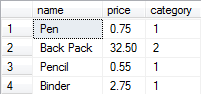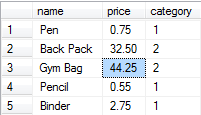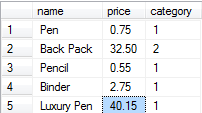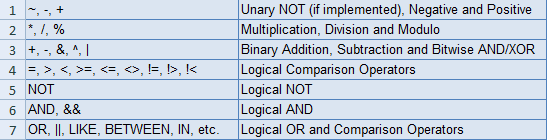# Effective SQL: Understanding Order of Operations

Published on
28,368 Points
17,768 Views
6 Endorsements
Last Modified:
Awarded
Editor's Choice
Community Pick

## Introduction

Hopefully the following mnemonic and, ultimately, the acronym it represents is common place to all those reading: Please Excuse My Dear Aunt Sally (PEMDAS). Briefly, though, PEMDAS is used to signify the order of operations being Parenthesis (or brackets), Exponents (or roots which can be expressed as a fractional exponent 1/n), Multiplication/Division and Addition/Subtraction.

If you have ever doubted how Math can be used in everyday life, then hopefully this is a good example. I think most forget when dealing with technology or challenges in general, it is all about approaching the situation with a definitive list of known truths and methodologies how to solve issues. You don't have to have the answers going in, just keep it simple and apply what you know like Math rules. SQL syntax is no different and so we will explore in this article how PEMDAS can aid programmers in avoiding some pitfalls or ensuring proper results through being very explicit about the order in which code is evaluated.

If you do this successfully, it should make even complex formulas in your SQL syntax easy to produce.

So let's get started...

During the editing of an article some months back, the topic of order of operations came up for discussion and there the data looked as similar to the below:
``````-------------+---------+---------
name         |price    |category
-------------+---------+---------
Pen          |0.75     |   1
Back Pack    |32.50    |   2
Gym Bag      |44.25    |   2
Pencil       |0.55     |   1
Binder       |2.75     |   1
-------------+---------+---------
``````
[step=""]««setup»»
To reproduce the above data, you can execute SQL similar to the below T-SQL example created for MS SQL Server.

(table structure -- create statement)
``````create table products(
name varchar(50),
price decimal(8,2),
category int
);
``````
(sample data -- insert statement)
``````insert into products(name, price, category)
values('Pen', 0.75, 1);

insert into products(name, price, category)
values('Back Pack', 32.50, 2);

insert into products(name, price, category)
values('Gym Bag', 44.25, 2);

insert into products(name, price, category)
values('Pencil', 0.55, 1);

insert into products(name, price, category)
values('Binder', 2.75, 1);
``````
[/step]
Now that we have some data, consider the results of this t-sql query:
``````select name, price, category
from products
where category = 1
or category = 2
and price <= 40.00
;
``````...and the results of this t-sql query:
``````select name, price, category
from products
where category = 2
or category = 1
and price <= 40.00
;
``````Why are these different?

I am guessing the title gives away the answer that this has to do with order of operations with respect to AND and OR operators. Before I address this, it is important to understand which result is correct ... to do so, the original intent must be clear. Clarity is the purpose of parenthesis or brackets, so let's start there.

## 1. Parenthesis

Using parenthesis aids in maintaining the order in which operators are evaluated in an equation or, in this case, logical operators in a conditional. Being in full control of order of operators ensures we achieve the given intent consistently.

And just to make this clearer, let's add the following data and re-look at query results 1-1:
``````insert into products(name, price, category) values('Luxury Pen', 40.15, 1);
``````If you re-run query 2, you will see the same results because what is happening is that we are getting all records in the first category listed and then only those in the second that are less than or equal to \$40.00 in cost.

So let's clarify...

(category is either 1 or 2 with a price <= 40)
``````(category = 1 or category = 2) and price <= 40.00
``````

(category is 1 or category is 2 with a price <= 40)
``````category = 1 or (category = 2 and price <= 40.00)
``````

The addition of the parenthesis around both category filters drastically changes things and makes syntax such as category in (1, 2) also valid, whereas, having category around the last two conditions of the where make it more deliberate that we get results shown in 1-1 illustrations.

A simpler case of this can be seen in the difference between mathematical results of:
``````select 10 - 2 + 5 from dual;
``````

...and...
``````select 10 - (2 + 5) from dual;
``````

Moral: Be clear. Keep it simple!

## 2. Expressions and Element-based Math

Expressions:
"Exponents" is what the E in PEMDAS stands for, but, in the computer world, exponents and roots are achieved through expressions.

For example, the POW or POWER function is used for creating exponents like 10^3 and would be expressed as:
``````select power(27, 3);
``````

In addition, you may have functions like SQRT (square root) or SQUARE as well as the ability of using negative or fractional exponentials with POW[ER] to achieve a given root:
``````select power(27, 1/3), power(27, 1.0/3), power(27.0, 1.0/3);
``````
Note: In MS SQL Server, the data type of the numbers involved in the POWER function matter. Therefore, the three expressions above yield three different results in Transact-SQL.

At the risk of veering too far off tangent, absolute value (e.g., |x|) is represented using expressions also. The correlation I like to think of is the ^ which can sometimes be used by us mathematical types to represent exponents (when superscript numbers are not available) and the | are both bitwise operators, which we will talk about later; therefore, the ^ cannot be used as the exponent operator.

Since functions contain parenthesis, you can consider that the operator precedence is maintained here as you evaluate everything inside the brackets before applying the formula of the expression itself.

This can be seen in practical use in my recent article on Conditional Aggregates. As exemplified by the percentage example query, the expressions will evaluate first so that later operators like multiplication and division have a value upon which to act.

Moral: Know your expressions and be creative in how you use them!

MSACC: http://www.techonthenet.com/access/functions/
MSSQL: http://msdn.microsoft.com/en-us/library/ms177516.aspx
MySQL: http://dev.mysql.com/doc/refman/5.5/en/mathematical-functions.html
PLSQL: http://www.techonthenet.com/oracle/functions/

Element-based Math:
Another "E" (as I am taking liberty of calling it element-based math) is for the priority given to unary operations: involving operators that act on a single element. Check your SQL systems implementation for specific unary operators available, but examples are: ~ (bitwise NOT), - (negative) and + (positive).

## 3. Multiplication (or Modulo) and Division then Addition and Subtraction

Multiplication/Modulo/Division:
These operators should be pretty self explanatory (except maybe Modulo), but as seen above when the proper order of operations is not considered, the results could be unexpected or inconsistent with the business intent. This is where it is best to be very explicit and possibly over utilize parenthesis in your syntax.

Subsequently, beyond assuring proper order of operations is clear, it is more efficient to simplify your mathematical formulas when possible thus eliminating multiple operations, potentially, to begin with.

For example, you may see an equation like the following where an interest rate stored as a whole number (e.g., 10%) is divided by and then multiplied by a principal column and a literal value (e.g., 5 for number of years):

``````select interest_rate / 100 * principal * 5
from loans;
``````

Reducing this formula to the below saves on extra processing by SQL:

``````select interest_rate * principal * 0.05 ...
``````

Additionally, as eluded to in the Conditional Aggregates article, this simplification of operations has just helped us avoid side effects of implicit type conversion to INT here when evaluating 5 / 100 which would normally come out to be 0 in MS SQL.

Addition/Subtraction:
As above, this is straight-forward, but just remember its order is after the above arithmetic operators if no parenthesis are used and you should try to simplify when possible so that you don't have literal values acting as operands when you can do the math offline and plug in the product, quotient, sum or difference directly into the query.

Moral: Do the math; check your answer!

## BONUS: Type Conversions

Consequently, it is good to note at this point, while on this train of thought (i.e., dealing with arithmetic operators), that you should take into consideration the type conversions and the order in which they occur naturally to ensure you understand their effect on your equation's results.

For example, note the difference in results of the same division problem from above, using explicit conversion:
``````convert(decimal(10,4), 5 / 100)
``````
``````convert(decimal(10,4), 5) / 100
``````

Going back to the Expressions section, the latter works since the CONVERT expression will occur first then the division by 100, changing this evaluation from the division of two Integers to normal decimal math (or from a different point of view forces an implicit conversion of the literal 100 in denominator to match the numerator). Note well that often doing these explicit conversions of operands can increase overall query performance.

## BONUS: Bitwise and Logical Operations

Bitwise Operations:
Circling back to the precedence order of addition/subtraction, the bitwise operators AND (&), OR (|) and Exclusive OR (^ or XOR) fall on equal plain; therefore, the importance is these operations will occur after multiplication/division but before logical operations. This fact ensures that you can do simple things like:
``````select name, price, category
from products
where price > category^2;
``````
Keeping in mind ^2 could have been any other operation above like *2 or +2.

Logical Operations:
``````2b OR NOT 2b
``````
...that is the question!

The NOT will be evaluated first here and then the connecting logical operator like the OR shown here. Following this, the AND operator has precedence and then the OR and other comparative statements similar to it (e.g., LIKE, IN, BETWEEN, ANY, ALL, SOME, etc.  -- check your SQL implementation for a more complete/correct list in your system).

One way to think of this is AND limits a condition or the number of records further as you are potentially dividing your already filtered recordset into smaller groups: ones that meet the additional criteria and the ones that don't. Along this same line of reasoning, OR can be likened to adding dissimilar rows to the same resultset. The key difference there is this doesn't equate to a straight addition of row counts as a single record may satisfy both conditions, so don't waste too much time trying to figure out why a < 5 which results in 10 rows combined with OR a < 3 which results in 5 rows doesn't equal 15 rows.

Eureka!

Now we understand the answer to our introductory question, right?
``````category = 2 or category = 1 and price <= 40.00
``````

Knowing what we know now, it should be clear that the above doesn't translate to category of 2 or 1 and ... but instead:
``````category = 2 or (category = 1 and price <= 40.00)
``````

## Conclusion

In summary, know your order of operations and utilize them in your everyday SQL to code more efficiently and effectively.

Let's recap:

Use parenthesis - a lot of the pitfalls folks get into with data calculations using SQL are due to a lack of explicit consideration for operator precedence and so later when code is innocently reorganized by another developer, the results can be drastically changed as we saw in flipping which category appeared first in our where condition in the introduction. With parenthesis, someone maintaining the original code would know for certain the intent and as such are likelier to not make such a change without consideration for its impact on the meaning of the query.
Expressions are evaluated next, so use this to your advantage for things like type conversion.
Keep the simple math and logical conditions just that: simple! As a reminder of the unary, binary and logical operators’ precedence, see the illustration below:Hope that helps...

Thanks as usual for reading!

Best regards and happy coding,

Kevin C. Cross, Sr. (mwvisa1)

=-=-=-=-=-=-=-=-=-=-=-=-=-=-=-=-=-=-=-=-=-=-=-=-=-=-=-=-=-=-=-=-=-=-=-=-=-=-=-=-=
If you found this article helpful, please click the Yes button after the question just below. This will give me (the author) a few points and might encourage me to write more articles.

If you didn't or otherwise feel the need to vote No, please first leave a comment to give me a chance to answer and perhaps to improve this article.

Thank you!
=-=-=-=-=-=-=-=-=-=-=-=-=-=-=-=-=-=-=-=-=-=-=-=-=-=-=-=-=-=-=-=-=-=-=-=-=-=-=-=-=
6
Ask questions about what you read
If you have a question about something within an article, you can receive help directly from the article author. Experts Exchange article authors are available to answer questions and further the discussion.
Get 7 days free